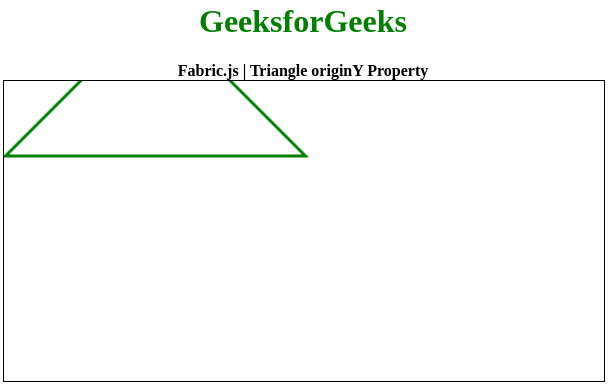# Fabric.js | Triangle originY Property

• Last Updated : 18 Aug, 2020

In this article, we are going to see how to set the vertical origin of the transformation of an object canvas triangle using FabricJS. The canvas triangle means the triangle is movable and can be stretched according to requirement. Further, the triangle can be customized when it comes to initial stroke color, height, width, fill color, or stroke width.
To make it possible we are going to use a JavaScript library called FabricJS. After importing the library, we will create a canvas block in the body tag that will contain the triangle. After this, we will initialize instances of Canvas and triangle provided by FabricJS and set the vertical transformation of the triangle using originY property and render the Triangle on the Canvas as given in the example below.
Syntax:

```fabric.Triangle({
width: number,
height: number,
originY: string
});
```

Parameters: This function accepts three parameters as mentioned above and described below:

• width: It specifies the width of triangle.
• height: It specifies the height of triangle.
• originY: It specifies the vertical transformation of a canvas triangle.

Example: This example uses FabricJS to set the vertical transformation of canvas triangle.

## javascript

 ```` ```    ````        ``Fabric.js | Triangle originY Property``    ````    ` `    ````    ````` ```    ``
``        ``

``            ``GeeksforGeeks ``        ``

``        `` ``            ``Fabric.js | Triangle originY Property ``        `` ``    ``
``    ````    ````    ` `    ````` ``

Output:My Personal Notes arrow_drop_up